PowerCustom SearchGraphical analysis of the basic circuitMatter, Energy, and ElectricityPower ratingPOWER

Power, whether electrical or mechanical, pertains to the rate at which work is being done. Work is done whenever a force causes motion. When a mechanical force is used to lift or move a weight, work is done. However, force exerted WITHOUT causing motion, such as the force of a compressed spring acting between two fixed objects, does not constitute work.

Previously, it was shown that voltage is an electrical force, and that voltage forces current to flow in a closed circuit. However, when voltage exists but current does not flow because the circuit is open, no work is done. This is similar to the spring under tension that produced no motion. When voltage causes electrons to move, work is done. The instantaneous RATE at which this work is done is called the electric power rate, and is measured in WATTS.

A total amount of work may be done in different lengths of time. For example, a given number of electrons may be moved from one point to another in 1 second or in 1 hour, depending on the RATE at which they are moved. In both cases, total work done is the same. However, when the work is done in a short time, the wattage, or INSTANTANEOUS POWER RATE, is greater than when the same amount of work is done over a longer period of time.

As stated, the basic unit of power is the watt. Power in watts is equal to the voltage across a circuit multiplied by current through the circuit. This represents the rate at any given instant at which work is being done. The symbol P indicates electrical power. Thus, the basic power formula is P = E x I, where E is voltage and I is current in the circuit. The amount of power changes when either voltage or current, or both voltage and current, are caused to change.

In practice, the ONLY factors that can be changed are voltage and resistance. In explaining the different forms that formulas may take, current is sometimes presented as a quantity that is changed. Remember, if current is changed, it is because either voltage or resistance has been changed.

Figure 3-9 shows a basic circuit using a source of power that can be varied from 0 to 8 volts and a graph that indicates the relationship between voltage and power.

The resistance of this circuit is 2 ohms; this value does not change. Voltage (E) is increased (by increasing the voltage source), in steps of 1 volt, from 0 volts to 8 volts. By applying Ohm's law, the current (I) is determined for each step of voltage. For instance, when E is 1 volt, the current is: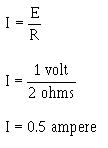Figure 3-9. - Graph of power related to changing voltage.Power (P), in watts, is determined by applying the basic power formula:and

P = E x I

P = 2 volts x 1 ampere

P = 2 wattsYou should notice that when the voltage was increased to 2 volts, the power increased from .5 watts to 2 watts or 4 times. When the voltage increased to 3 volts, the power increased to 4.5 watts or 9 times. This shows that if the resistance in a circuit is held constant, the power varies directly with the SQUARE OF THE VOLTAGE.

Another way of proving that power varies as the square of the voltage when resistance is held constant is: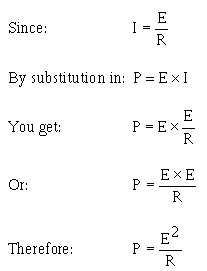Another important relationship may be seen by studying figure 3-10. Thus far, power has been calculated with voltage and current (P = E x I), and with voltage and resistance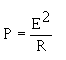Referring to figure 3-10, note that power also varies as the square of current just as it does with voltage. Thus, another formula for power, with current and resistance as its factors, is P = I2R. This can be proved by: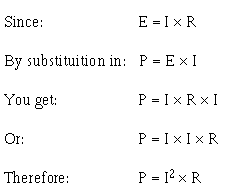Figure 3-10. - Graph of power related to changing current.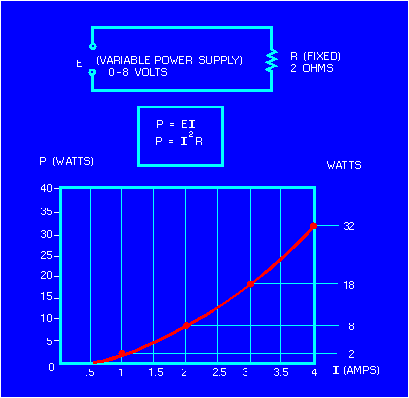Up to this point, four of the most important electrical quantities have been discussed. These are voltage (E), current (I), resistance (R), and power (P). You must understand the relationships which exist among these quantities because they are used throughout your study of electricity. In the preceding paragraphs, P was expressed in terms of alternate pairs of the other three basic quantities E, I, and R. In practice, you should be able to express any one of these quantities in terms of any two of the others.

Figure 3-11 is a summary of 12 basic formulas you should know. The four quantities E, I, R, and P are at the center of the figure. Adjacent to each quantity are three segments. Note that in each segment, the basic quantity is expressed in terms of two other basic quantities, and no two segments are alike.

Figure 3-11. - Summary of basic formulas.For example, the formula wheel in figure 3-11 could be used to find the formula to solve the following problem:

A circuit has a voltage source that delivers 6 volts and the circuit uses 3 watts of power. What is the resistance of the load?

Since R is the quantity you have been asked to find, look in the section of the wheel that has R in the center. The segmentcontains the quantities you have been given. The formula you would use is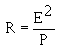The problem can now be solved.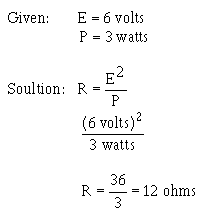What is the term applied to the rate at which a mechanical or electrical
force causes motion?
How can the amount of current be changed in a circuit?
What are the three formulas for electrical power?Integrated Publishing, Inc. - A (SDVOSB) Service Disabled Veteran Owned Small Business